# 6.2.1 Integers, PT3 Practice

Question 1:
Evaluate 5 + (–7)

Solution: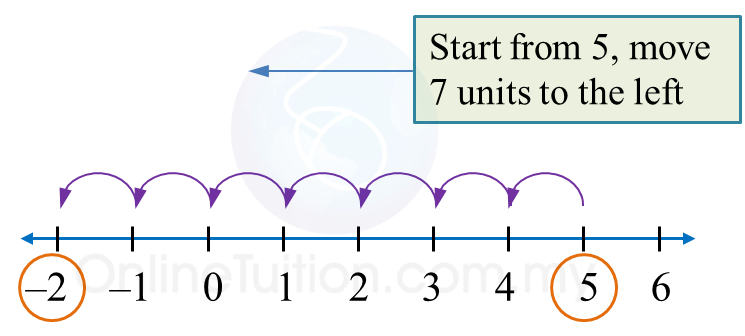Therefore,
5 + (–7) = 5 – 7
= –2

Question 2:
Evaluate –6 – (–5)

Solution:Therefore,
–6 – (–5) = –6 + 5
= –1

Question 3:
Simplify –3 + 7 + (–2)

Solution:Therefore,
–3 + 7 + (–2) = 4 + (–2)
= 4 – 2
= 2

Question 4:
Simplify 5 + (–4) + (–3)

Solution: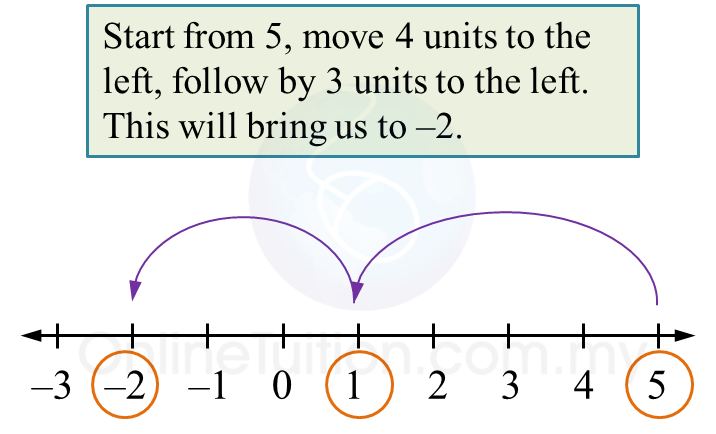Therefore,
5 + (–4) + (–3)
= 5 – 4 – 3
= –2

# 6.2 Integers

6.2 Addition and Subtraction of Integers

1.
Addition of integers can be done on a number line.
(a) To add a positive integer:
Move to the right (positive direction) on the number line.

(b)
Move to the left (negative direction) on the number line.2. Integers
with the same signs are called integers with like signs.
Example:
2 and 7, –25 and –5.

3. Integers with the different signs are called integers with unlike signs.
Example:
+2 and –7, –25 and 5.

Example 1:

Solve the following.
(a) 3 + (+4)
(b) 2 + (–5)

Solution:
(a)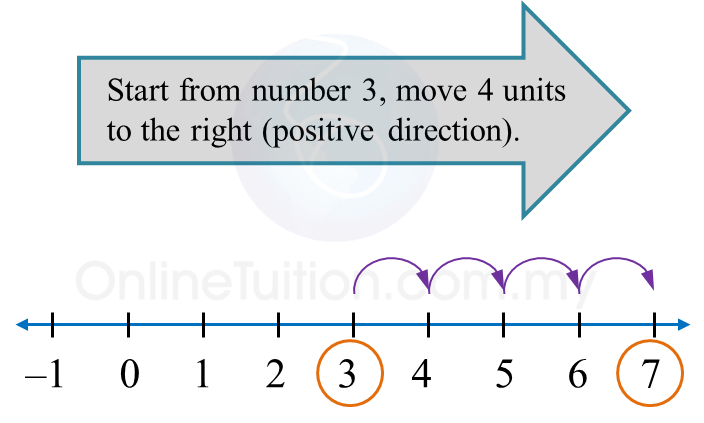Therefore,
3 + (+4) = 3 + 4
= 7

(b)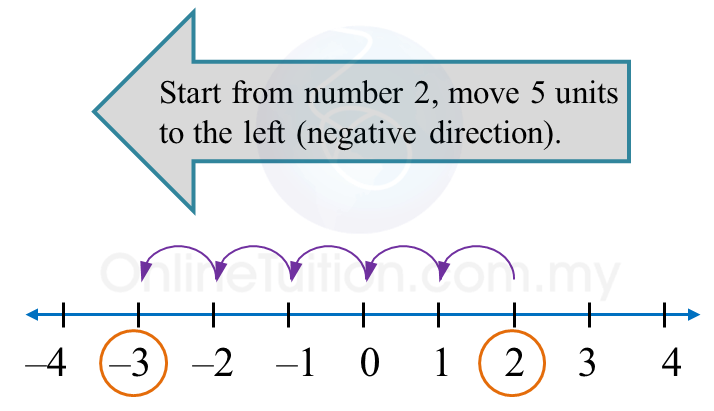Therefore,
2 + (–5)
= –3

6.2.3 Multiplication and Division of Integers
1. Multiplication and division of like signs gives (+)

$\overline{)\begin{array}{l}\text{}\left(+\right)×\left(+\right)=+\text{}\left(+\right)÷\left(+\right)=+\text{}\\ \text{}\left(-\right)×\left(-\right)=+\text{}\left(-\right)÷\left(-\right)=+\end{array}}$

2.
Multiplication and division of unlike signs gives ()

$\overline{)\begin{array}{l}\text{}\left(+\right)×\left(-\right)=-\text{}\left(+\right)÷\left(-\right)=-\text{}\\ \text{}\left(-\right)×\left(+\right)=-\text{}\left(-\right)÷\left(+\right)=-\end{array}}$

Example:
(a) –25 ÷ 5 = –5
(b)   8 × (–5) = –40

# 5.2.2 Percentages, PT3 Practice

Question 6:
Liza had 360 apples. She sold ⅝ of the apples. Then she gave 27 of the remaining apples to her neighbour.
Calculate the percentage of apples that Liza has left.

Solution:

Question 7:
Mei Ling took part in a science quiz competition. She answered 12 questions correctly. She answered 25% of the questions incorrectly.
Find the total number of questions in the quiz.

Solution:

Question 8:
Diagram below shows the prices of two items.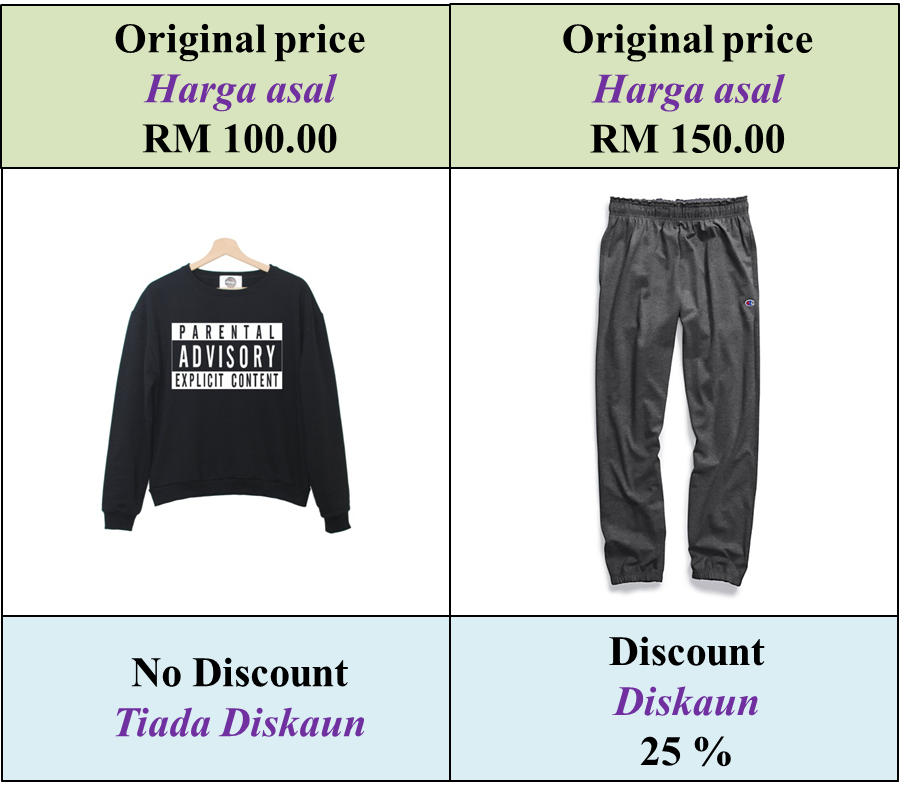Kenny buys a pair of track pants and 2 pieces of sweaters.
Calculate the total amount Kenny has to pay.

Solution:

Question 9:
Table below shows the prices and the discounts for the same brand of school bag sold at four shops, P, Q, R and SWhich shop offers the cheapest price?

Solution:

Question 10:
Table below shows dividend received by Janice from her investment.Janice reinvests RM204 from the total dividends she received into ASB investment.
What is the remaining percentage that she did not use in her reinvestment?

Solution:

# 5.2.1 Percentages, PT3 Practice

Question 1:
Rahman bought a house at RM180 000. The market price of the house increases by 15 % per year. After a year, Rahman sold his house at the price of RM 205 000.
Is the selling price higher compared to the market price? Show your working.

Solution:

Question 2:
A company makes a profit of RM 50 000 in year 2016. The projection of profit increase is fixed at 20 % from the previous year.
Predict the company profit in year 2018.

Solution:

Question 3:
Sharon wants to buy a new car with the price of RM 78 500. Her father gives her some money, which is 30 % of the car price. The deposit for the car is $\frac{1}{5}$ of the price.
Would her father’s contribution be enough for her deposit? Give your reason with calculation.

Solution:

Question 4:
The profit of Company P and Company Q in March are RM38 000 and RM48 000 respectively. In April, the profit of Company P has increased by 20%.
Given the profit for both companies are equal in April, find the percentages decrease in profit of Company Q.

Solution:

Question 5:
A biscuit making company needs 200 tonnes of flour every month. Factory K supplies 80 tonnes, while the rest is supplied by Factory M. In a particular month, Factory K faces a problem which forces it to reduce its output by 15%.
Find the total percentage of the flour output of Factory M in order to accommodate the requirement of the biscuit making company.

Solution: